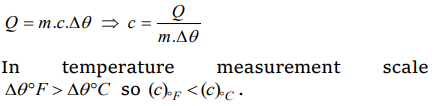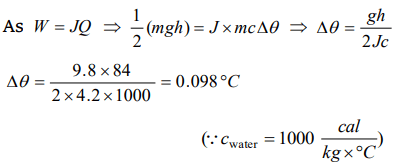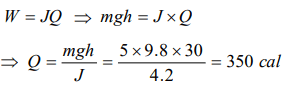## Thermal Properties of Matter Questions and Answers Part-10

1. If temperature scale is changed from °C to °F, the numerical value of specific heat will
a) Increases
b) Decreased
c) Remains unchanged
d) None of the above

Explanation:2. By exerting a certain amountof pressure on an ice block, you
a) Lower its melting point
b) Make it melt at 0°C only
c) Make it melt at a faster rate
d) Raise its melting point

Explanation: Increasing pressure lowers melting point of ice

3. When we rub our palms they gets heated but to a maximum temperature because
a) Heat is absorbed by our palm
b) Heat is lost in the environment
c) Produced of heat is stopped
d) None of the above

Explanation: Work done changes into heat energy, when the temperature of palm becomes above the atmosphere so it starts losing heat to the surroundings

4. A bullet moving with a uniform velocity v, stops suddenly after hitting the target and the whole mass melts be m, specific heat S, initial temperature 25°C, melting point 475°C and the latent heat L. Then v is given by
a) $mL=mS\left(475-25\right)+\frac{1}{2}.\frac{mv^{2}}{J}$
b) $mS\left(475-25\right)+mL=\frac{mv^{2}}{2J}$
c) $mS\left(475-25\right)+mL=\frac{mv^{2}}{J}$
d) $mS\left(475-25\right)-mL=\frac{mv^{2}}{2J}$

Explanation:5. A water fall is 84 metres high. If half of the potential energy of the falling water gets converted to heat, the rise in temperature of water will be
a) 0.098°C
b) 0.98°C
c) 9.8°C
d) 0.0098°C

Explanation:6. A body of mass 5 kg falls from a height of 30 metre. If its all mechanical energy is changed into heat, then heat produced will be
a) 350 cal
b) 150 cal
c) 60 cal
d) 6 cal

Explanation:7. In supplying 400 calories of heat to a system, the work done will be
a) 400 joules
b) 1672 joules
c) 1672 watts
d) 1672 ergs

Explanation: W = JQ = 4.18 * 400 = 1672 joule

8.The weight of a person is 60 kg. If he gets 105 calories heat through food and the efficiency of his body is 28%, then upto how much height he can climb (approximately)
a) 100 m
b) 200 m
c) 400 m
d) 1000 m

Explanation:9. The temperature of Bhakhra dam water at the ground level with respect to the temperature at high level should be
a) Greater
b) Less
c) Equal
d) 0°C

10. The height of a waterfall is 84 metre. Assuming that the entire kinetic energy of falling water is converted into heat, the rise in temperature of the water will be$\left(g=9.8 m\diagup s^{2}, J =42 joule \diagup cal\right)$